Browse by Topic

# Wolfram|Alpha, Now in Español!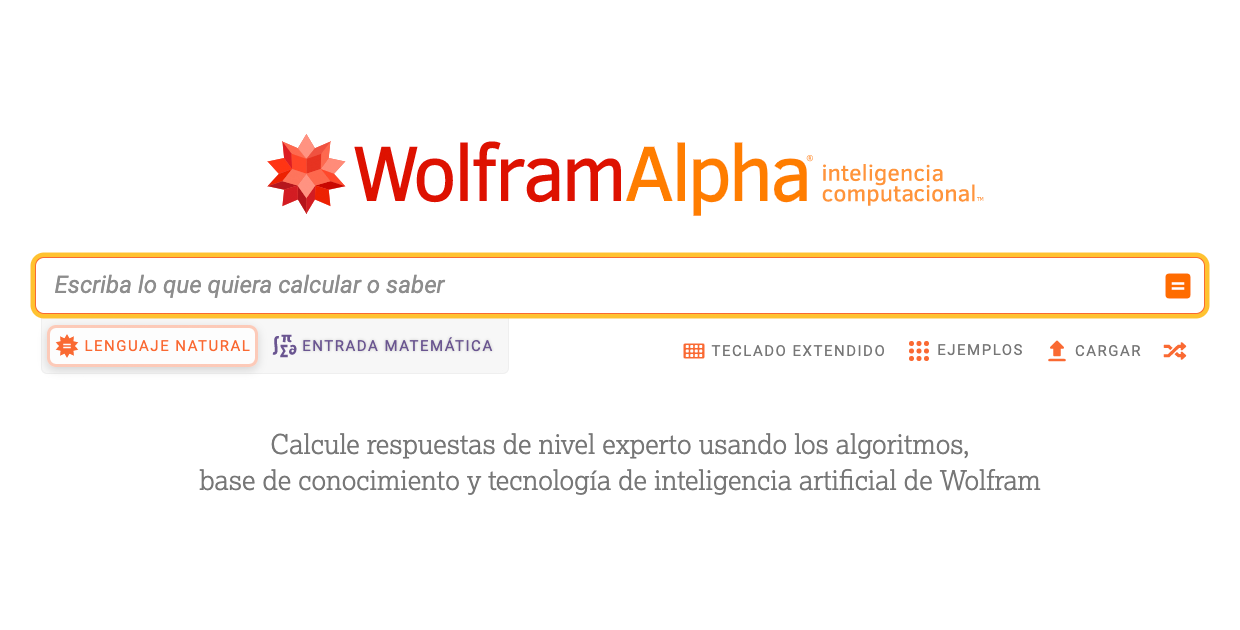We are happy to announce that Wolfram|Alpha, the world’s first computational knowledge engine, is now available in Spanish.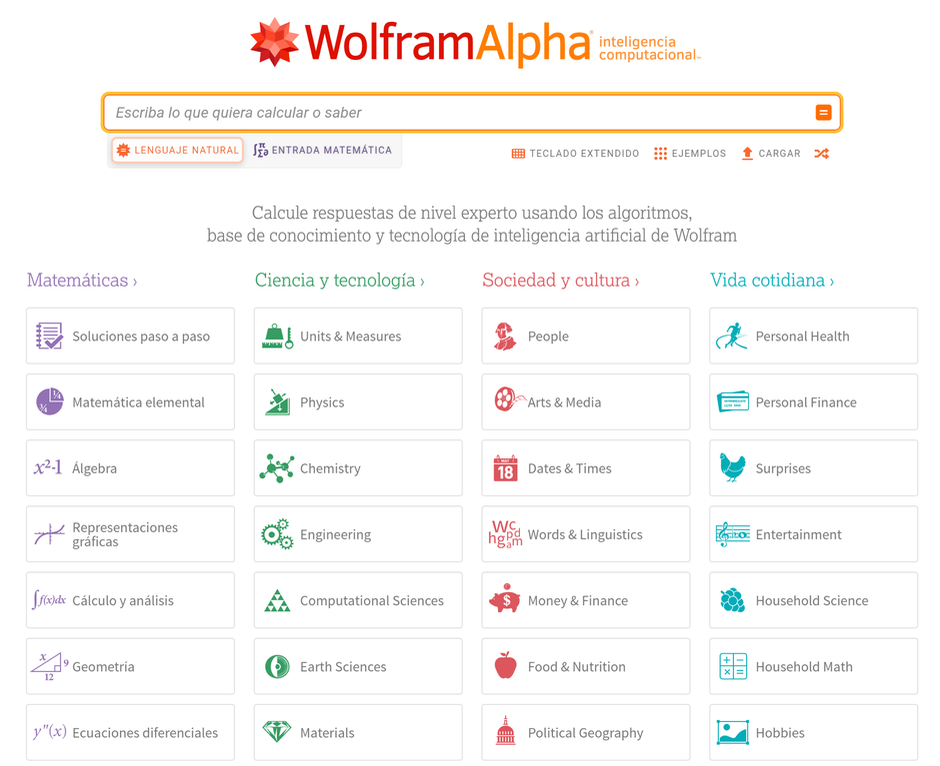✕

## What Is Wolfram|Alpha?

Wolfram|Alpha is a computational knowledge engine that understands and answers questions in natural language. It is based on four key components:

• Natural language processing: to understand the questions asked by users in the most intuitive way.
• A curated database: terabytes of data curated by experts that covers a large range of topics.
• Dynamic computation: once the question is interpreted, Wolfram|Alpha extracts the relevant information from its database and uses a collection of tens of thousands of algorithms to summarize and generate new knowledge.
• Extensive answers: Wolfram|Alpha not only returns the exact answer to the user, but also additional information for a better understanding.

Wolfram|Alpha is developed with Mathematica and the Wolfram Language, the programming language whose powerful symbolic programming capabilities enable the mathematic and algorithmic processing of questions and results.

Wolfram|Alpha in Spanish is not simply a translation of the English content, but an adaptation to Spanish of the natural language rules and result generation.

## What Can You Do with Wolfram|Alpha in Spanish?

The Spanish version includes all the math topics available in the English version. The wide diversity of math topics included in Wolfram|Alpha allows it to answer almost any question, from elementary math to differential equations and elliptic functions.

We can explore Wolfram|Alpha by simply making a query, clicking the Random button or visiting the large example gallery, which is classified by topic.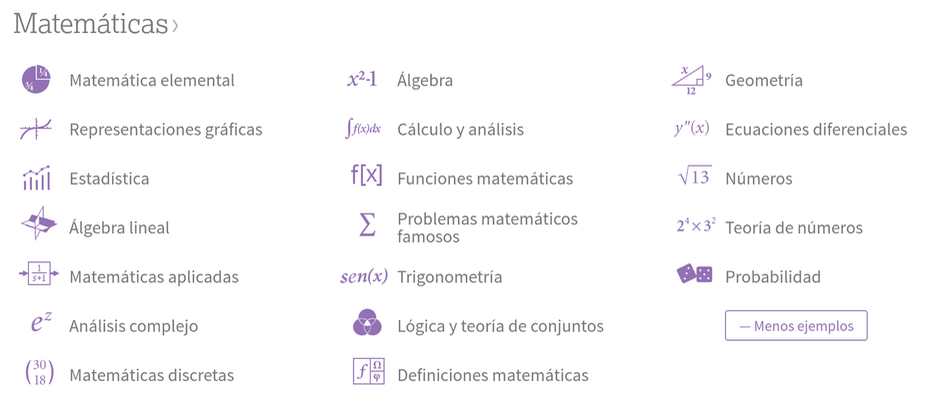✕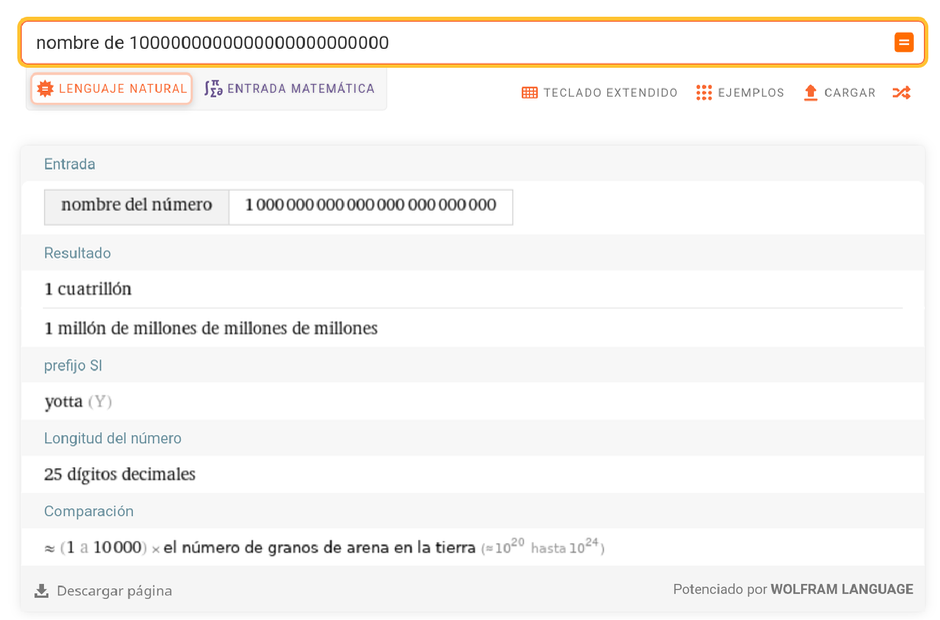✕

Wolfram|Alpha not only understands the question and gives the answer, it also returns related information.

Algebra may seem like a hard topic, but Wolfram|Alpha will obtain the solution to almost any equation you can write.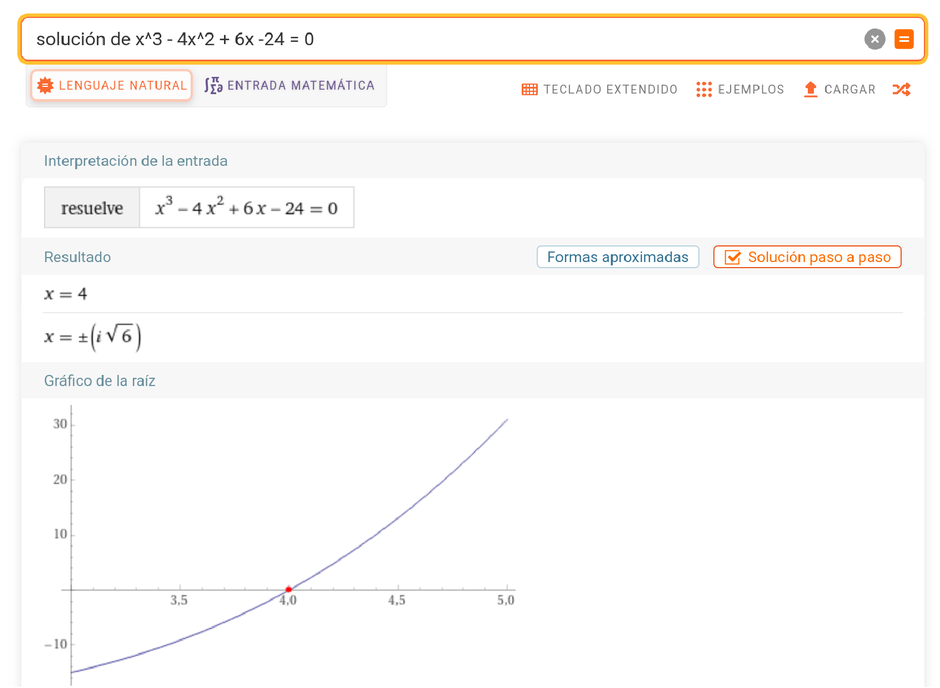✕

Geometry is a very important math topic, and Wolfram|Alpha’s vast knowledge allows it to compute the properties of geometric figures, simple or complex.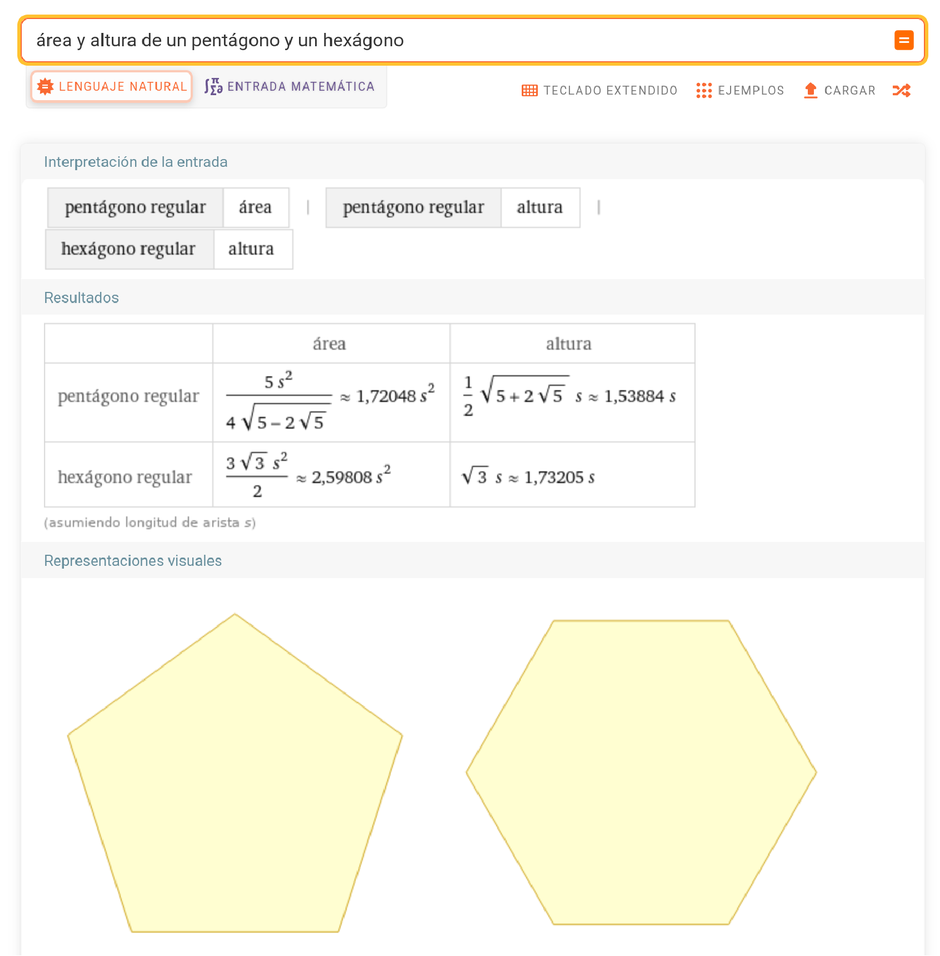✕

Do you want to know about integrals? You can ask about “integrales que contienen cos(u)” (“integrals that contain cos(u)”) and get a large gallery of examples of integrals.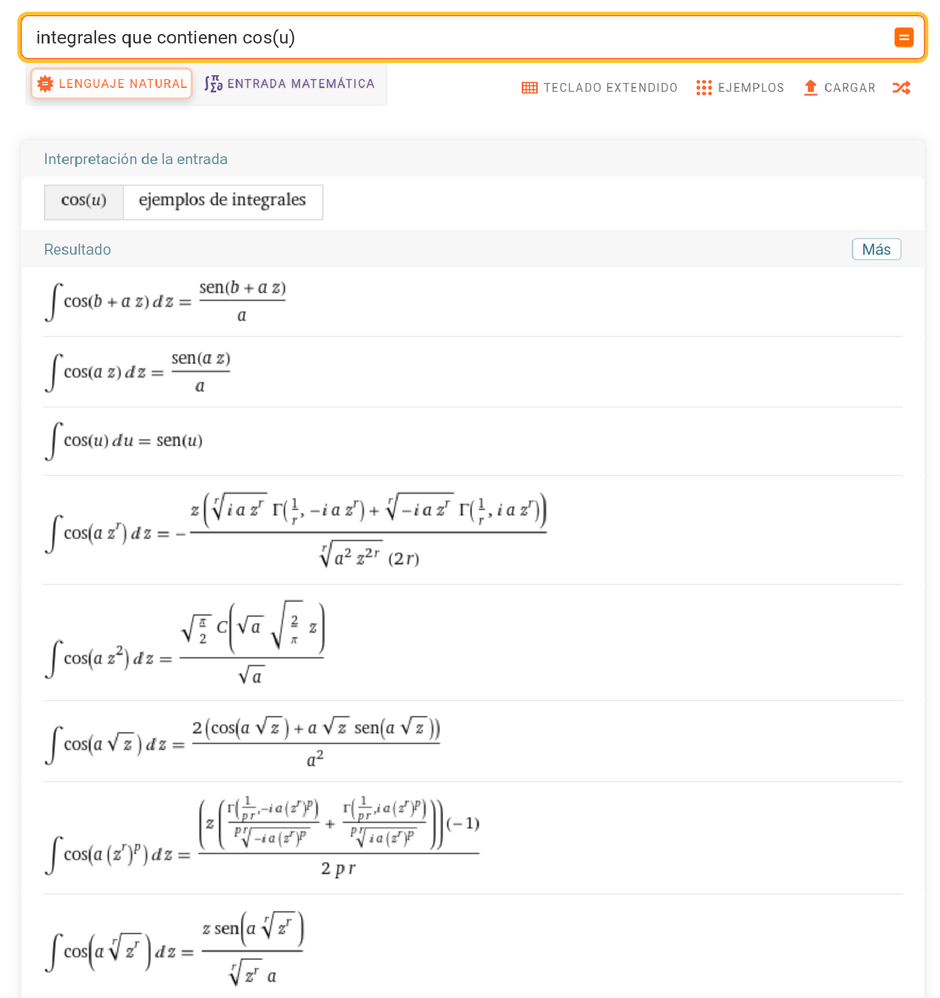✕

Or if you need to solve a complicated differential equation, you can simply write it and get the solution.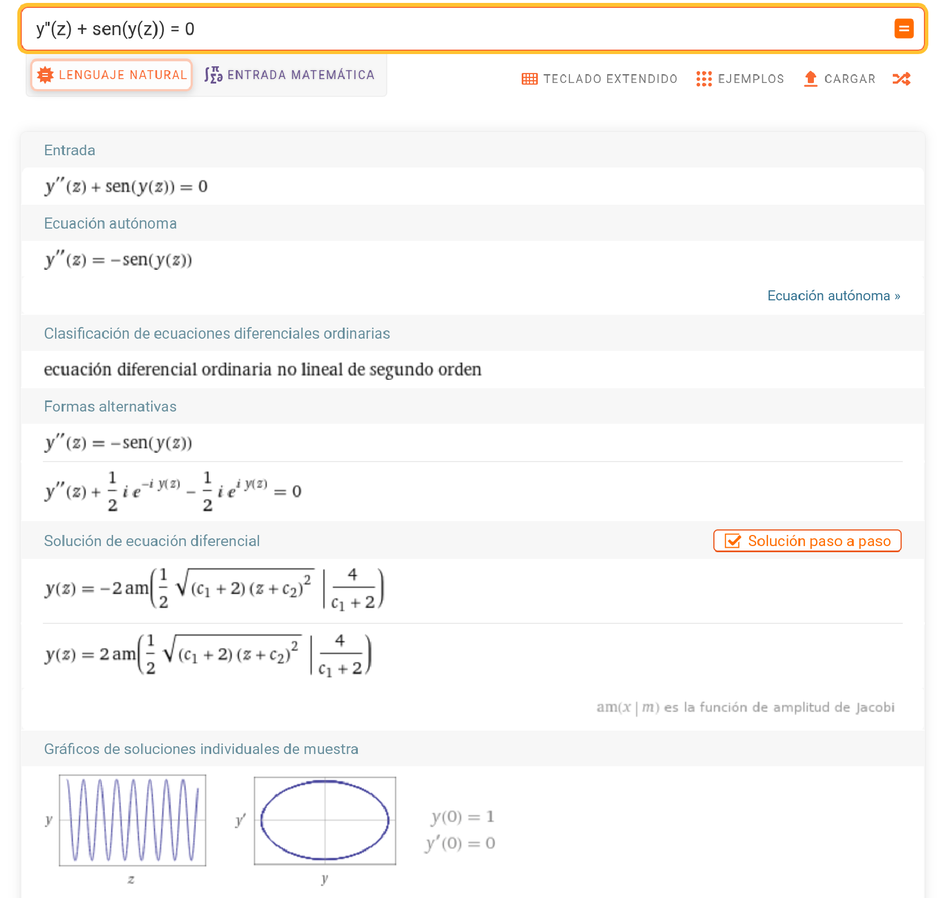✕

Moreover, if we need more details, we can ask for the step-by-step solution. Here’s a sample.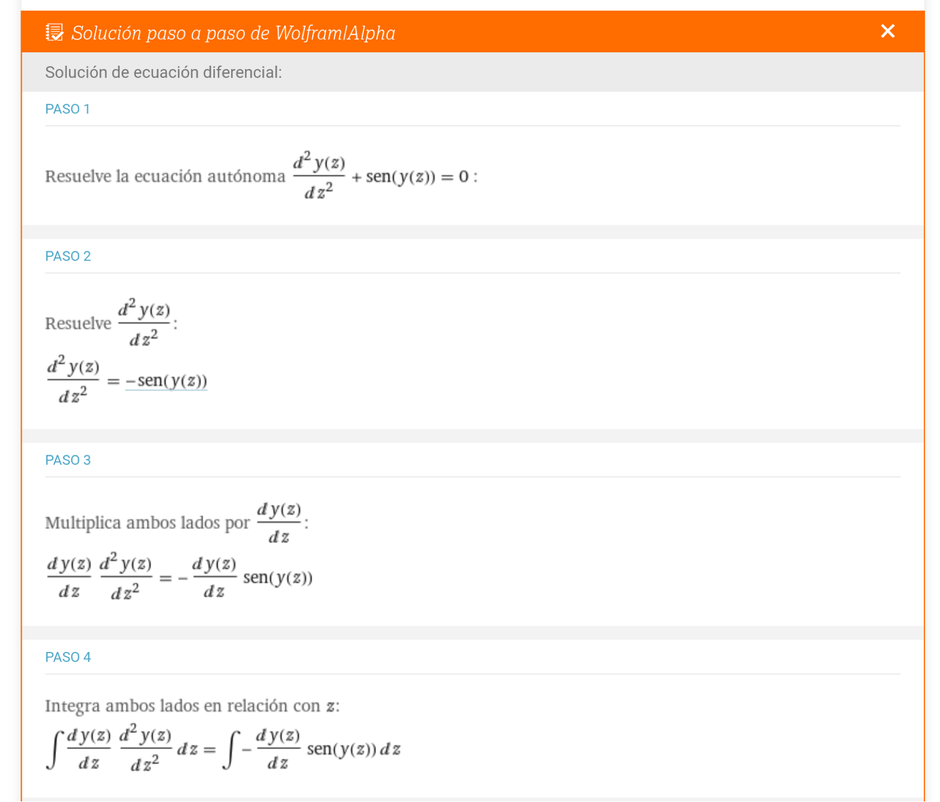✕

It can also answer applied math questions, like this cover problem.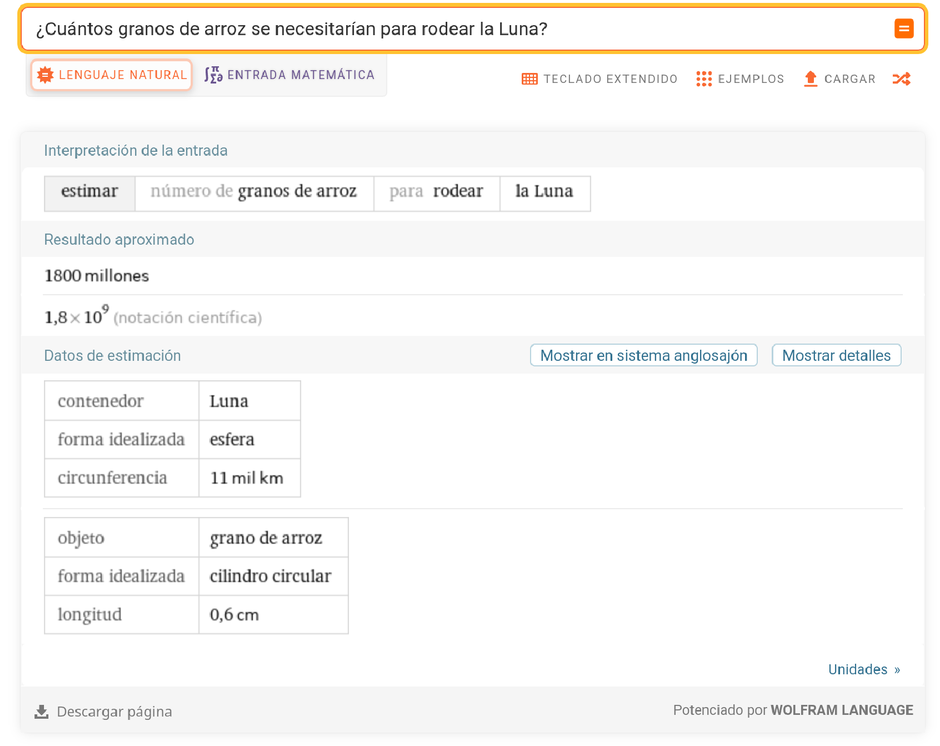✕

There are many more topics that can be explored in Wolfram|Alpha in Spanish—be sure to try it!✕